Instant Maths Help:

# Edexcel A Level

## Straight Lines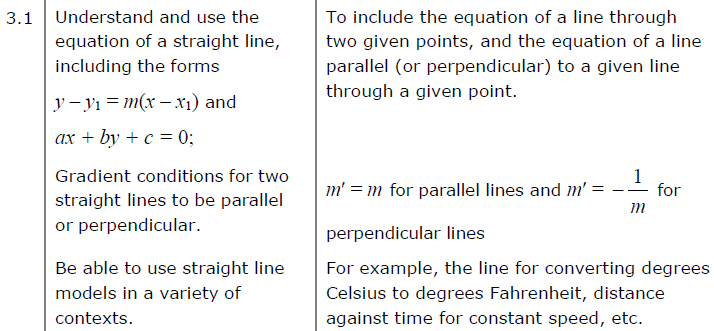##### Edexcel 8MA0/01 Jun 2023 AS Exam Q. 10 :   8 marks in 9:36 min.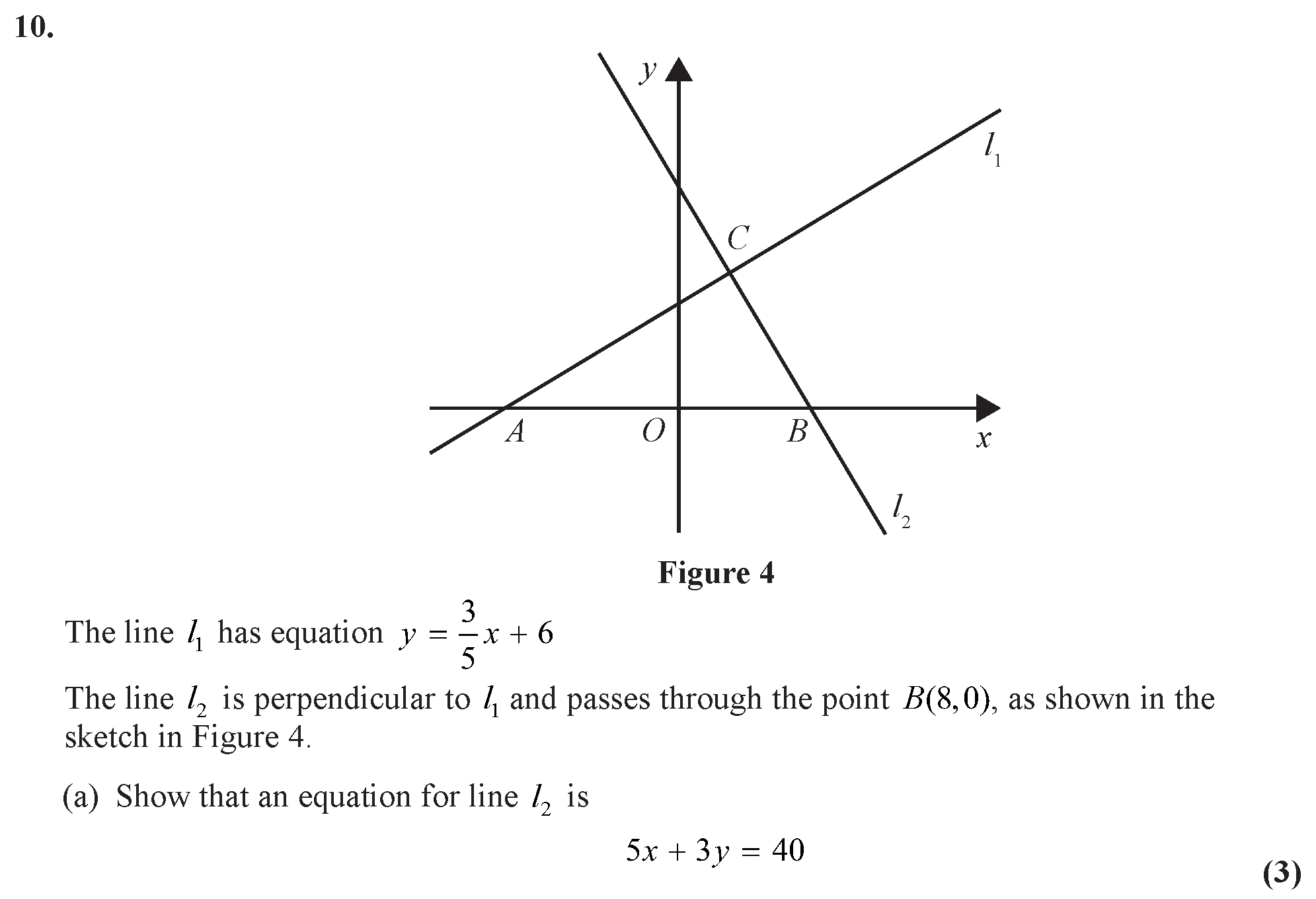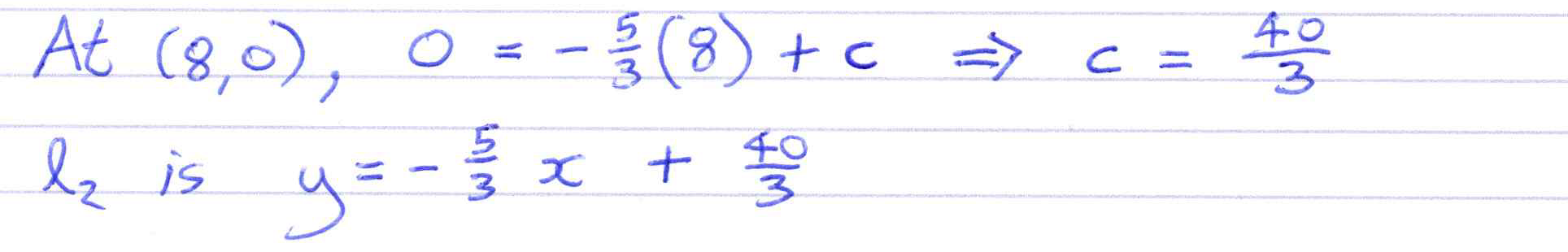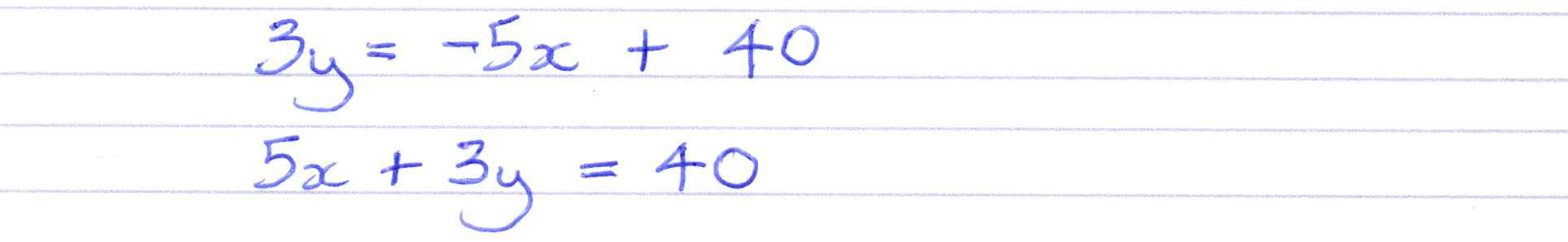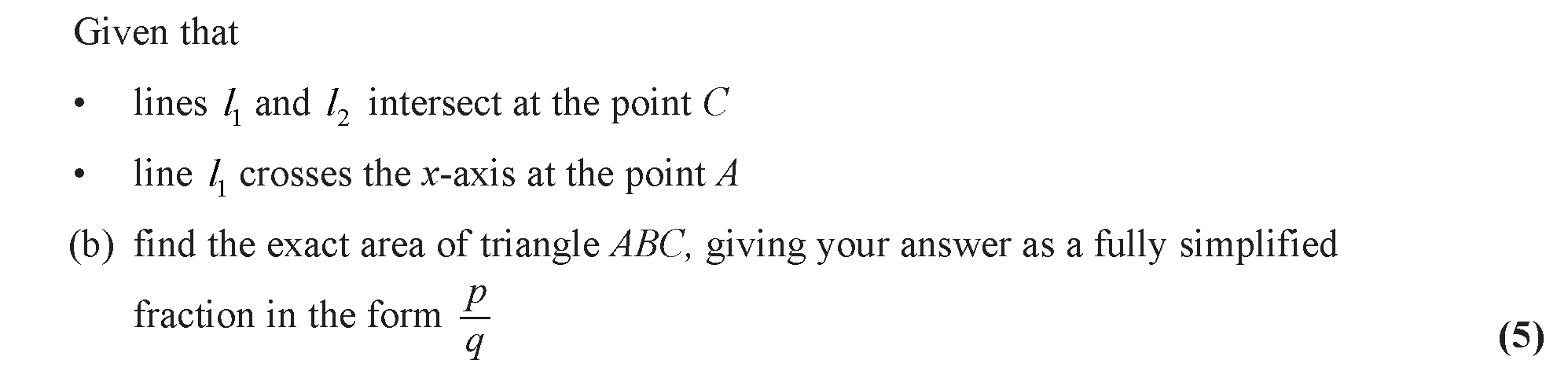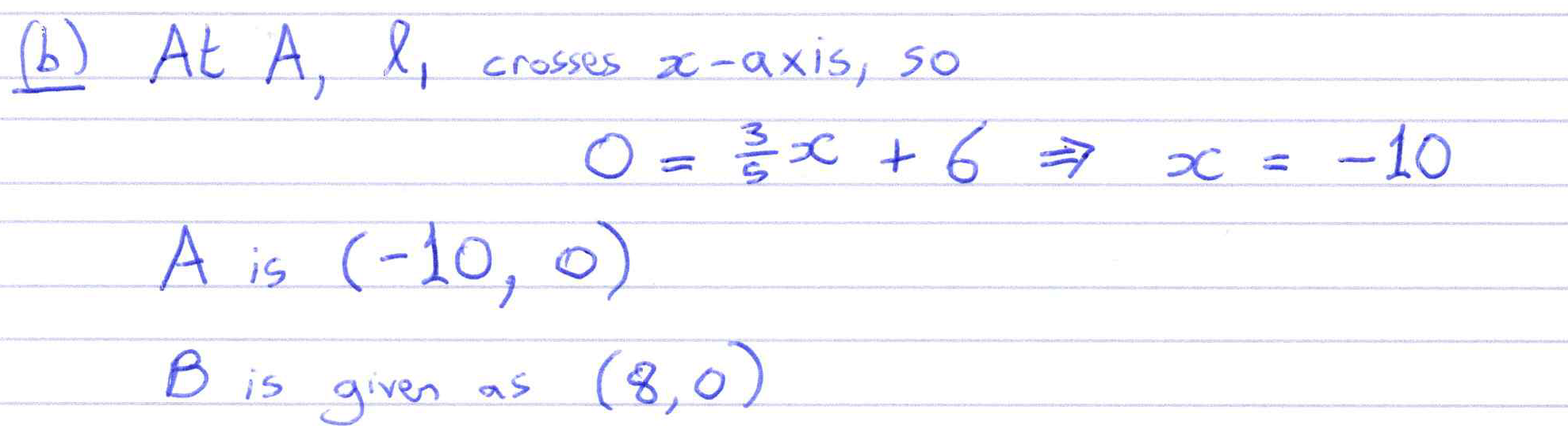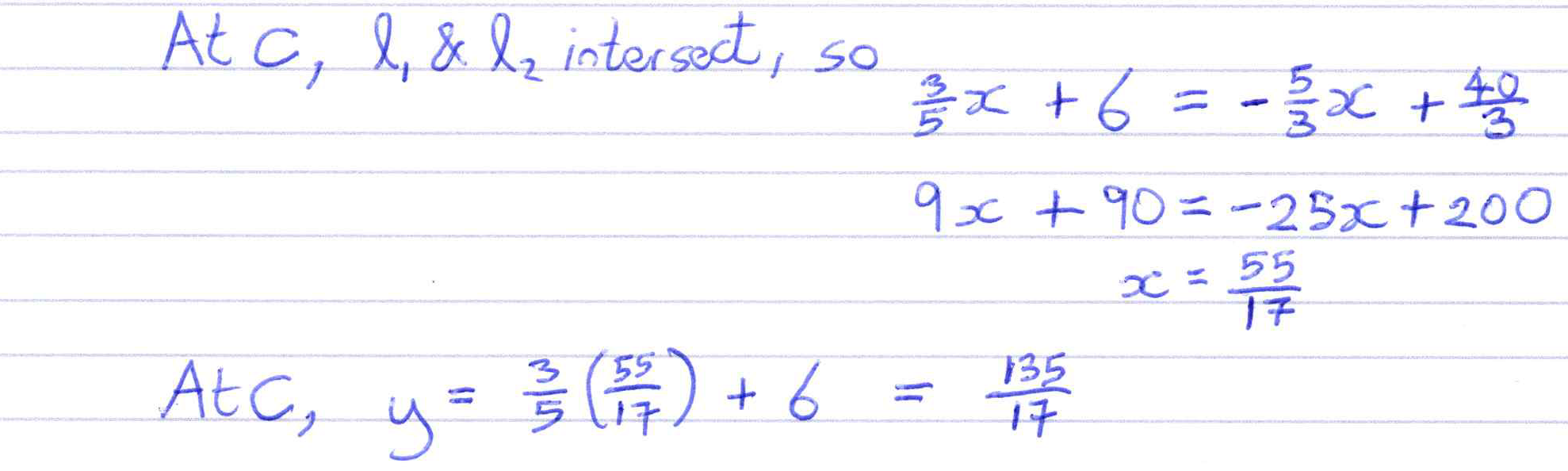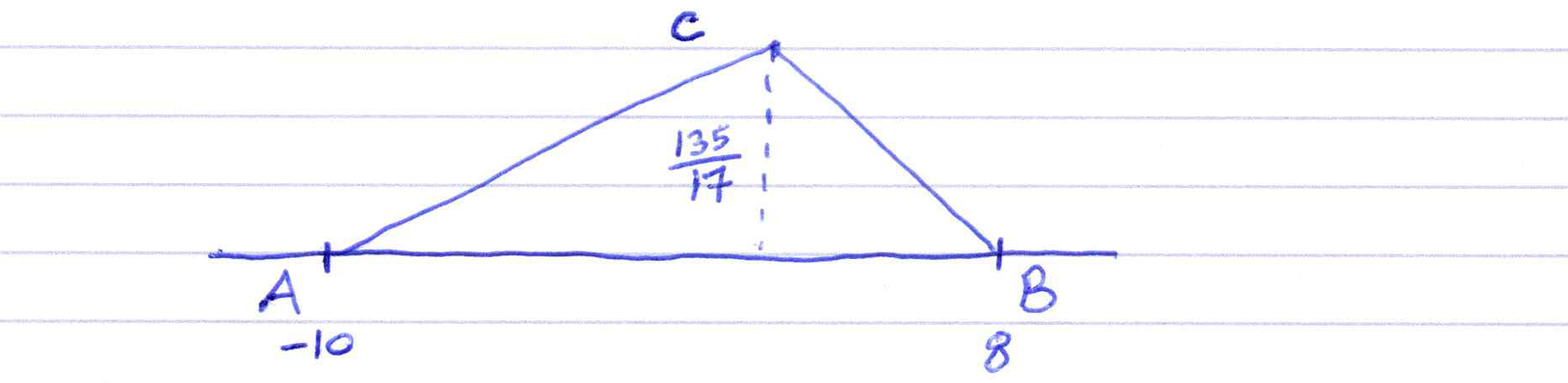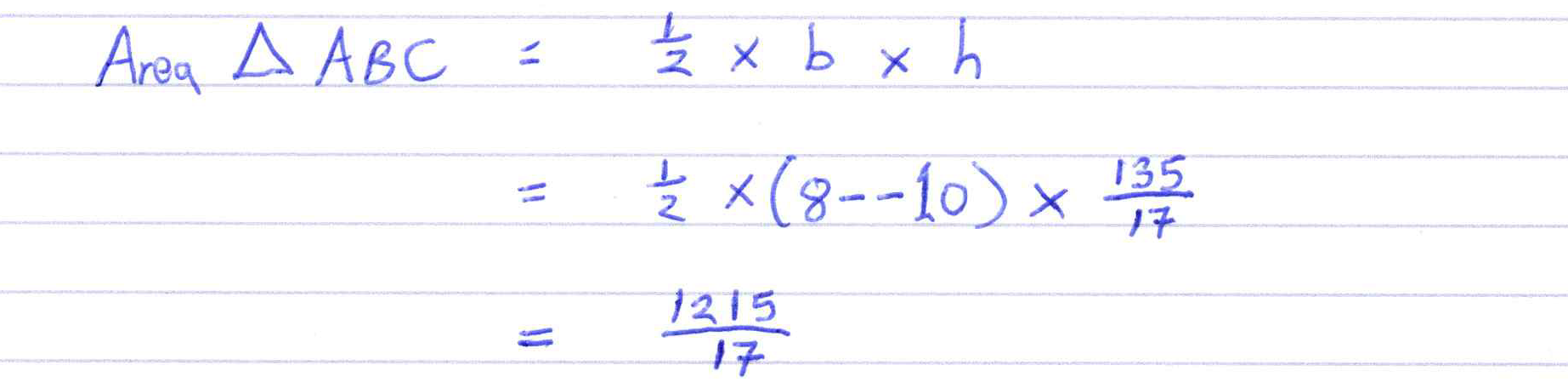##### Edexcel 8MA0/01 Jun 2023 AS Exam Q. 7 :   8 marks in 9:36 min.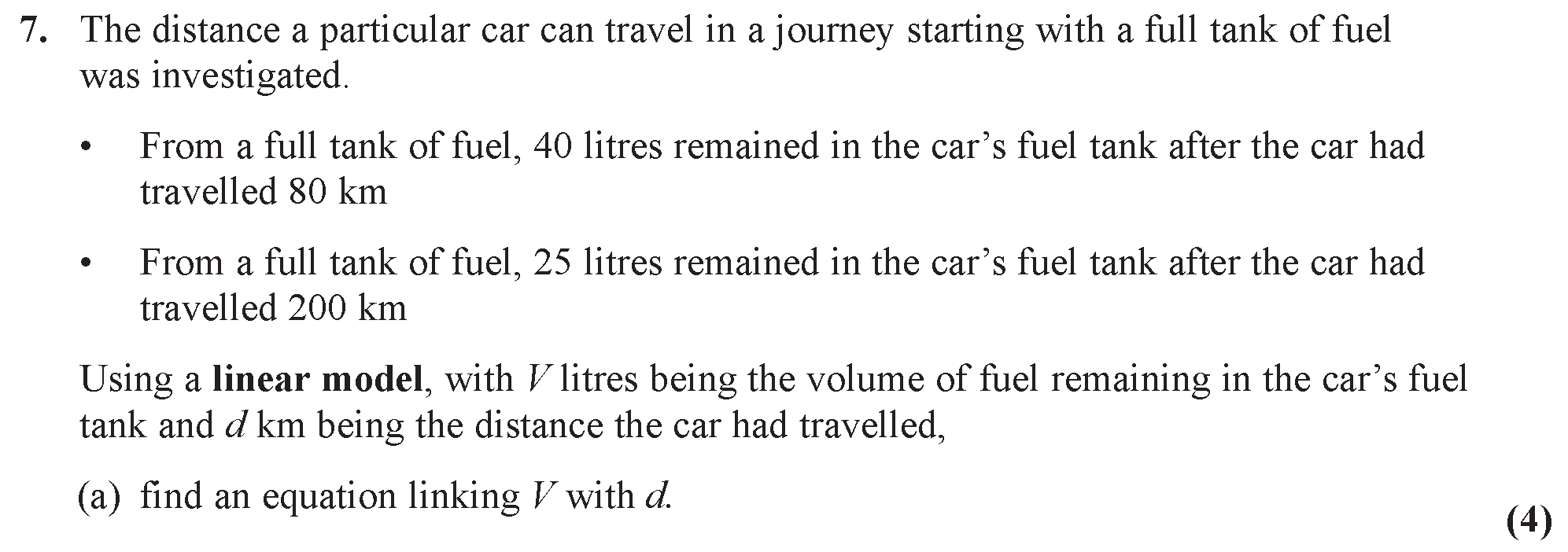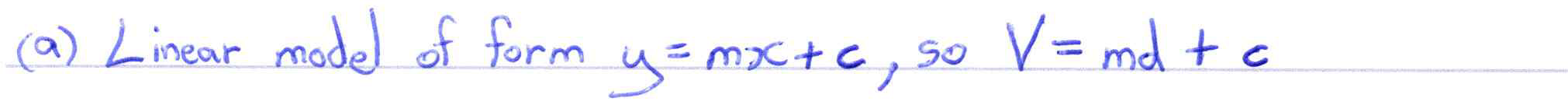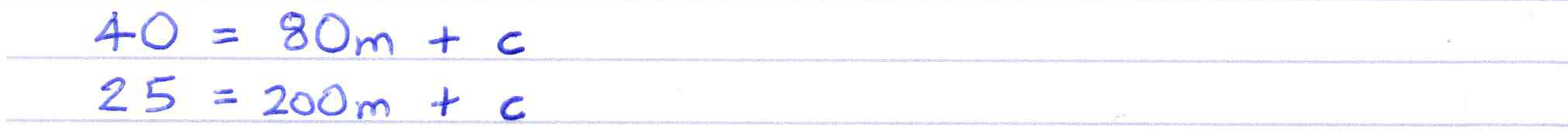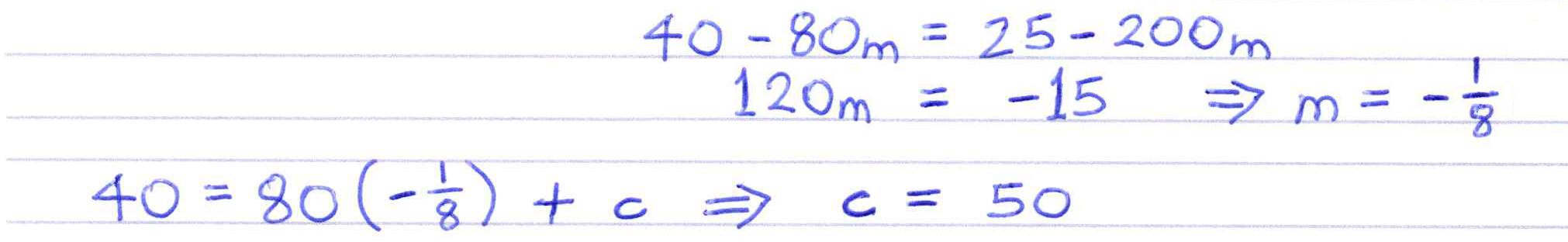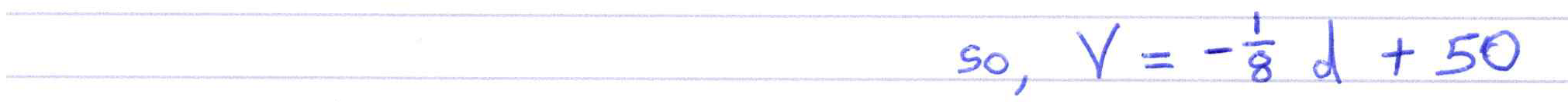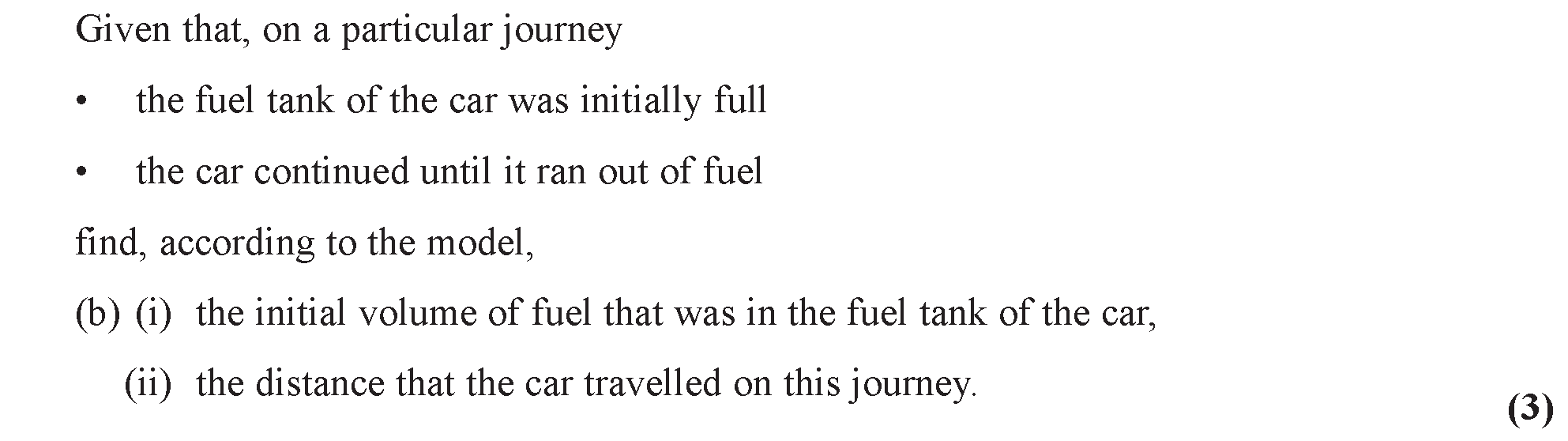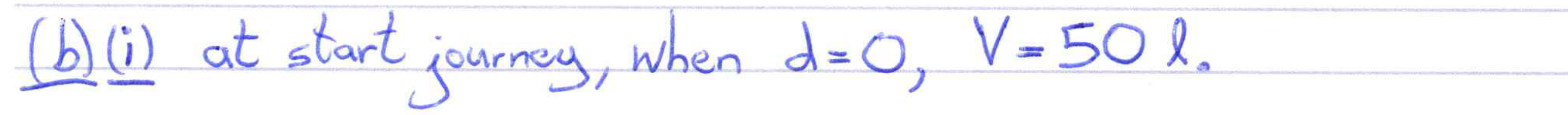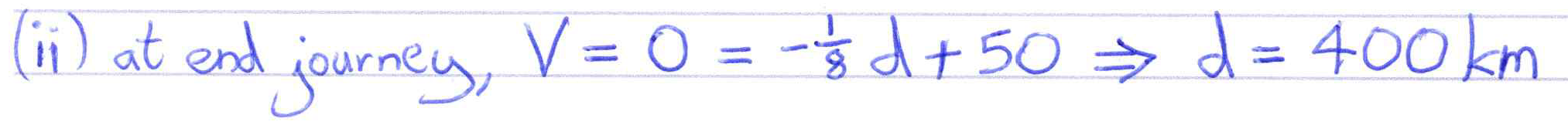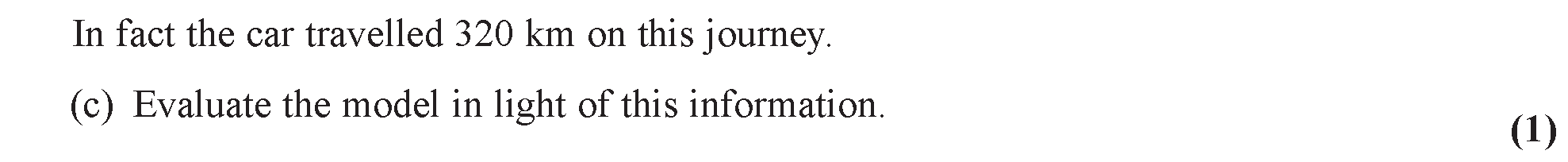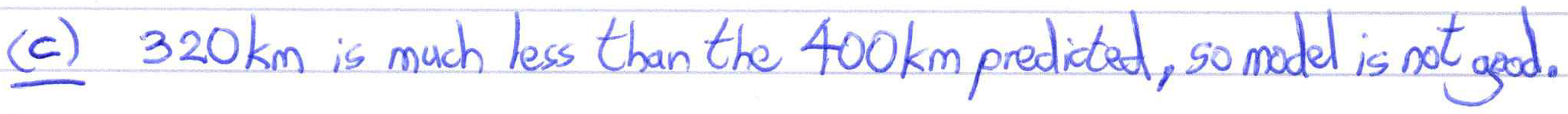##### Explore gradient between two points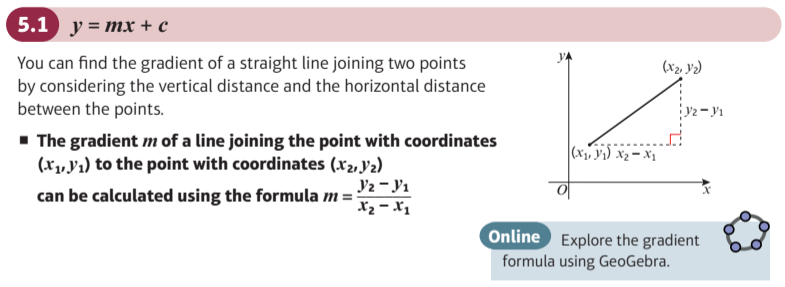##### Explore gradient through a point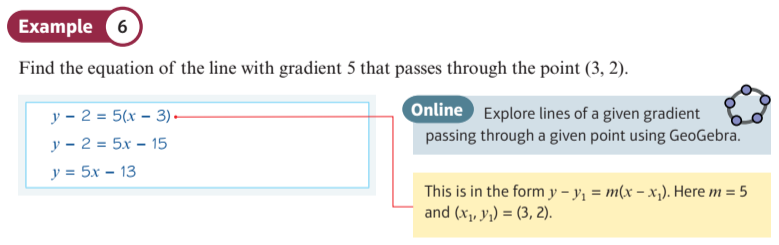##### Straight lines: Page 95, Example 8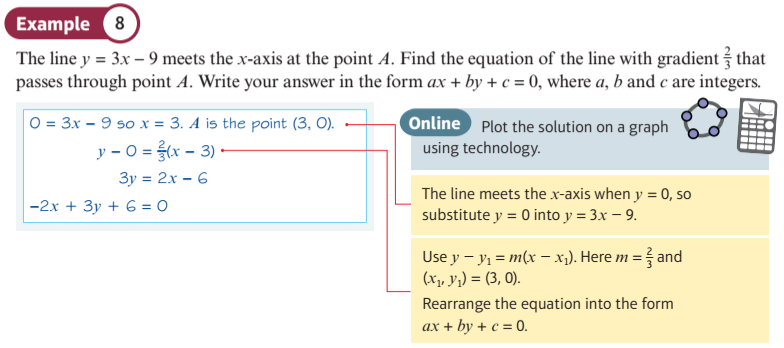##### Straight lines: Page 98, Example 11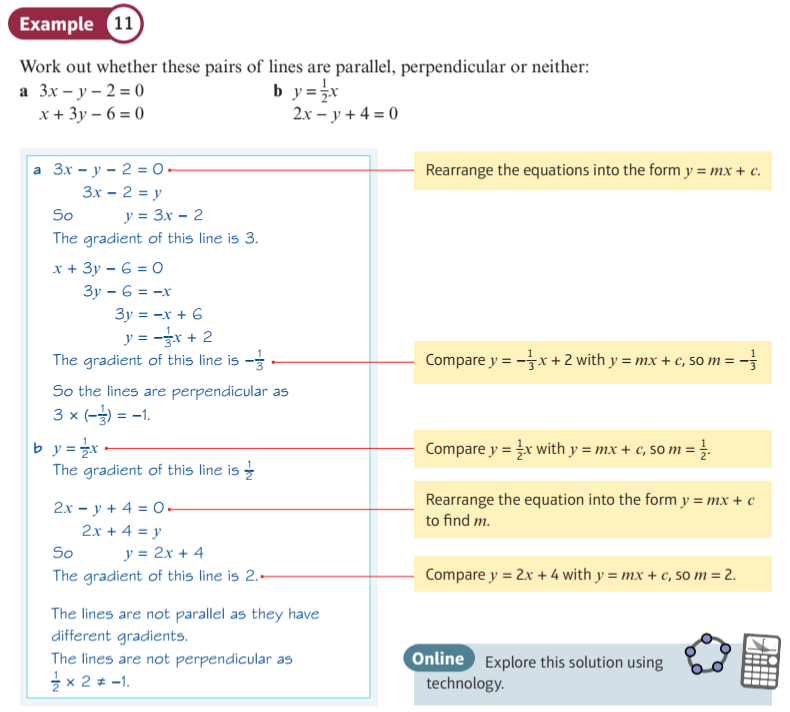##### Straight lines: Page 101, Example 14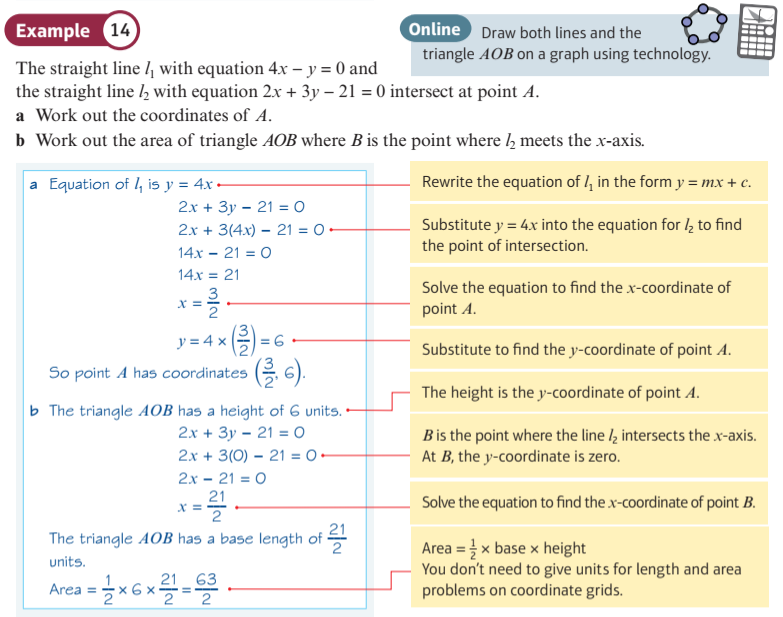##### Matching Lines 1
Each example shows some points on a straight line, but with at least one point wrongly placed. Use the sliders to plot the correct lines, and see the mistakes highlighted.
##### Matching Lines 2
You can select eight green lines on this page. Using the sliders to match the blue line to the green line will reveal the equation in each case, but can you say what the equation is before making the match?
##### Matching Lines 3
You can select ten green lines on this page. Using the sliders to matching the blue line to the green line will reveal the equation in each case, but can you say what the equation is before making the match?
##### Line as ax + by = c
Use the display below to investigate straight lines with equations of the form $\color{blue}{ax + by = c}$.